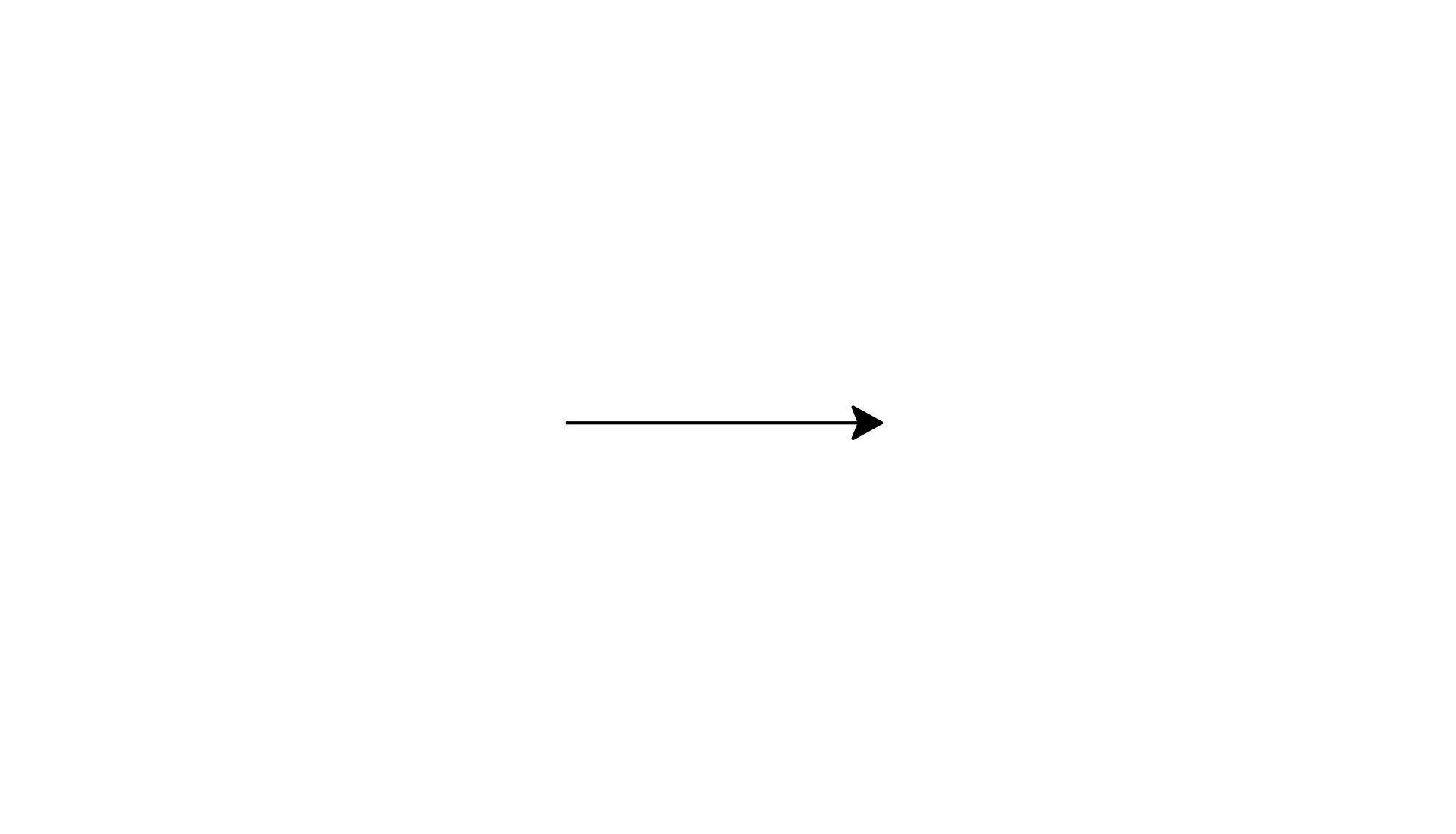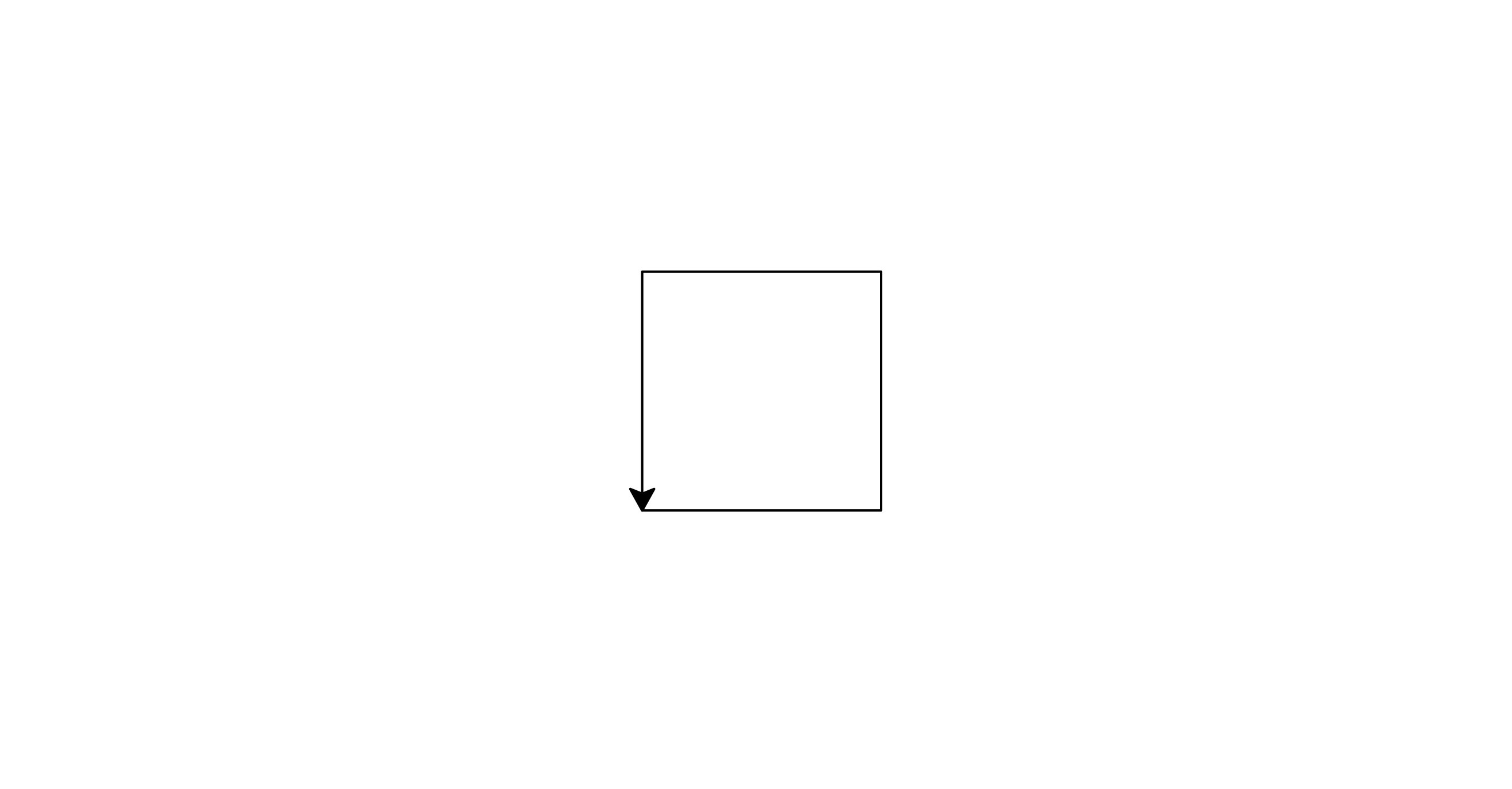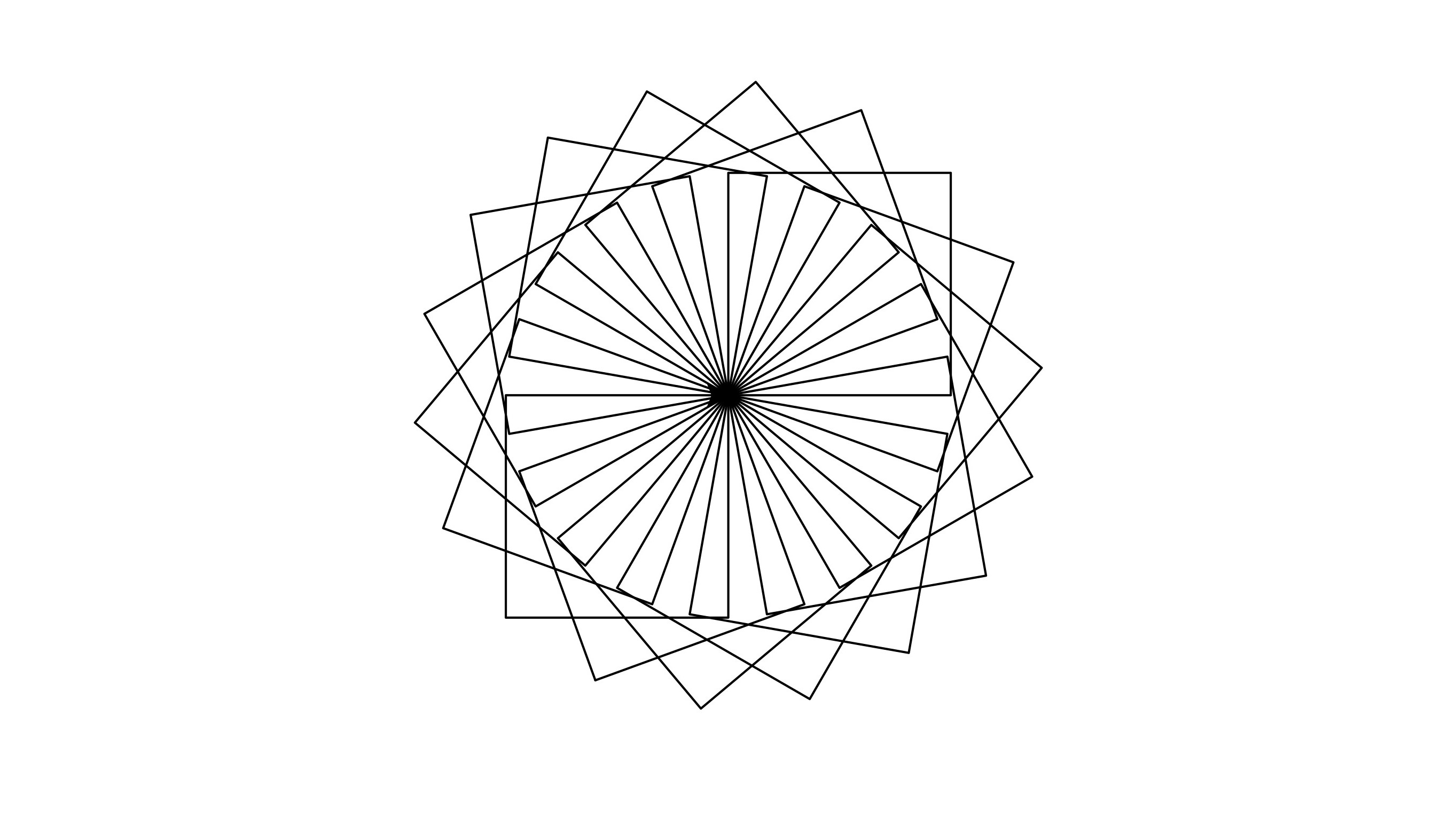# Day 10: Turtle

## Starting Turtle Library¶

At first we have to import turtle library. In order to import turtle library we will simply use import turtle.

import turtle
sandeep = turtle.Turtle()
sandeep.forward(100)
# In the end we have to write
turtle.done()


The program creates a turtle named sandeep. It starts with the origin $(0,0)$ and moves toward the right $100px$. The image looks as followsSo basically turtle traces a path according to given instruction.

Similar to forward, we can use the following functions to control our drawing according to the name.

• Forward: used as sandeep.forward(100) to move $100px$ in forward direction.
• Backward: used as sandeep.backward(100) to move $100px$ in backward direction.
• Left: used as sandeep.left(60) to turn $60^\circ$ towards left.
• Right: used as sandeep.right(60) to turn $60^\circ$ towards right.

We can directly go to a specified coordinate using goto function as sandeep.goto(100, 100)

### 1. Creating a Square¶

We can use these basic knowledge to draw a square as follows.

import turtle
sandeep = turtle.Turtle()
sandeep.forward(100)
sandeep.left(90)
sandeep.forward(100)
sandeep.left(90)
sandeep.forward(100)
sandeep.left(90)
sandeep.forward(100)
# In the end we have to write
turtle.done()We can see that there is a loop in the program, so we can use loop to make it short.

import turtle
sandeep = turtle.Turtle()
for i in range(4):
sandeep.forward(100)
sandeep.left(90)
# In the end we have to write
turtle.done()


Now we can use function to create a square and then use that function to create more complicated shapes based on square.

import turtle
sandeep = turtle.Turtle()
def square():
for i in range(4):
sandeep.forward(100)
sandeep.left(90)

for i in range (18):
square()
sandeep.left(20)
# In the end we have to write
cv = turtle.getcanvas()
cv.postscript(file="file_name.ps", colormode='color')
turtle.done()